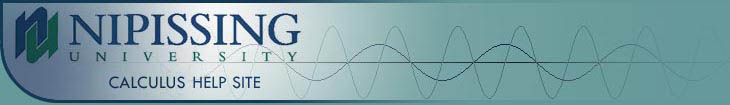TESTS
TESTS HOME

ONLINE TESTS
PRELIMINARY TESTS
GENERAL TESTS
ILRN WEBSITE

LIMITS
DERIVATIVES
RELATED RATES & OPTIMIZATION
CURVE SKETCHING
INTEGRALS
AREA & VOLUME
INVERSE FUNCTIONS

MAIN
HOME
TESTS
TUTORIALS
SAMPLE PROBLEMS
COMMON MISTAKES
STUDY TIPS
GLOSSARY
CALCULUS APPLICATIONS
MATH HUMOUR

## INVERSE FUNCTIONS TEST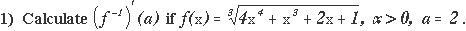a. 1 / 2
b. 4 / 7
c. 0
d. 12 / 17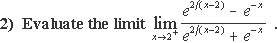a.b. 0
c. -d. 1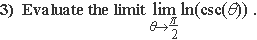a.b. 0
c. -d. 1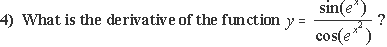a.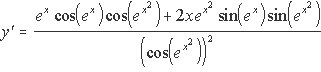b.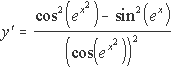c.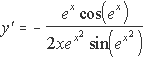d.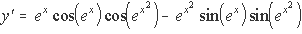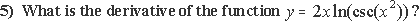a. y' = 2(ln(csc(x2)-2x2csc(x2)cot(x2))
b. y' = 4x2/ csc(x2)
c. y' = 2x / csc(x2 )
d. y' = 2(ln(csc(x2))-2x2cot(x2))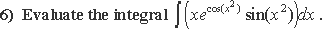a.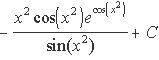b.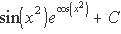c.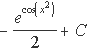d.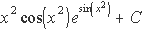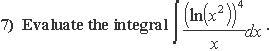a. (ln(x2))5 / 5x2 + C
b. (ln(x2))5 / 10 + C
c. ln(x7) / 5x2 + C
d. ln(x7) / 10 + C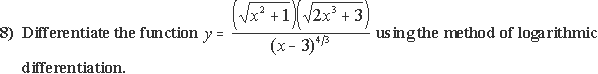a.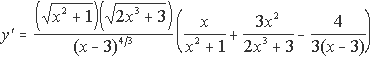b.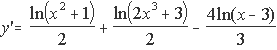c.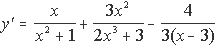d.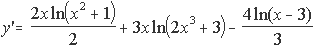If you had any trouble with this advanced test, it is strongly recommended that you review the content of the Inverse Functions Tutorial before attempting to take this test again. It is also recommended that you complete the General Inverse Functions Test on the iLrn website and the questions from the Inverse Functions Sample Problems page. The links for all of these resources can be found below.

Inverse Functions Tutorial
Inverse Functions Problems
General Inverse Functions Test on iLrn

|Top of Page |

COURSE HOMEPAGES
MATH 1036
MATH 1037

FACULTY HOMEPAGES
Alex Karassev
Ted Chase